In: Chemistry

# A beaker containing 50.0 ml of 0.300M Ca2+ at ph 9 is titrated with 0.150M EDTA....

A beaker containing 50.0 ml of 0.300M Ca2+ at ph 9 is titrated with 0.150M EDTA. Calculate the pCa2+ at the equivalence point

## Solutions

##### Expert Solution

Consider a complex formation reaction: Ca 2+ + EDTA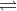[ CaY ] 2-

For above reaction, conditional formation constant is K' f = (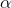Y 4-) K f

Therefore, K' f = 0.041 x 10 10.65 = 1.83 x 10 9

First , we Calculate  of volume of EDTA required to reach equivalence point

From above reaction , we have 1 mol Ca 2+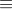1 mol  EDTA

Therefore, M ca 2+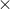V Ca2+ = M EDTAV EDTA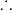V EDTA = M ca 2+V Ca2+ / M EDTA =

V EDTA = 0.300 M50.0 ml / 0.150 M

V EDTA = 100 ml

volume of EDTA required to reach equivalence point = 100 ml

At the equivalence point, all the calcium ions are consumed by added EDTA. Hence, at this stage concentration of Ca 2+ will be due to dissociation of [CaY] 2-.

Moles of [ CaY ] 2- = moles of EDTA added to the solution

No. of moles of EDTA added in the solution = 0.150 mol / L0.100 L = 0.015 mol

Volume of solution at equivalence point = Volume of Ca 2+ solution + volume of EDTA solution

Volume of solution at equivalence point = 50.0 + 100 ml =150.0 ml = 0.150 L

[CaY] 2-. = 0.015 mol / 0.150 L = 0.100 M

Let’s use ICE table.

 Concentration ( M ) Ca 2+ EDTA [CaY] 2- I 0.100 C +X +X -X E X X 0.100 - X

We have , K' f = [CaY2-] / [Ca 2+] [EDTA]

Therefore,K' f = 0.100 -X / (X)(X) = 1.8310 9

0.100 -X / (X)(X) = 1.8310 90.100  -X / X 2 = 1.8310 9

At equilibrium X is negligible as compared 0.100. Then we can write 0.100 / x 2 = 1.83 x 10 9X 2 = 0.100 / 1.8310 9 =5.46410 -11X = 7.39210 - 6 M[Ca 2+] = 7.93210 - 6 M

We have relation, pCa 2+ = - log [Ca 2+]pCa 2+ = - log 7.93210 - 6 = 5.13

ANSWER : pCa 2+ at equivalence point = 5.13

## Related Solutions

##### A 50.0-mL solution containing Ni2+ and Zn2+ was treated with 25.0 mL of 0.0452 M EDTA...
A 50.0-mL solution containing Ni2+ and Zn2+ was treated with 25.0 mL of 0.0452 M EDTA to bind all the metal. The excess unreated EDTA required 12.4 mL of 0.0123 M Mg2+ for complete reaction. An excess of the reagent 2,3-dimercapto-1-propanol was then added to displace the EDTA from zinc. Another 29.2 mL of Mg2+ was required for reaction with the liberated EDTA. Calculate the molarity of Ni2+ and Zn2+ in the original solution
##### You are titrating 110.0 mL of 0.050 M Ca2 with 0.050 M EDTA at pH 9.00....
You are titrating 110.0 mL of 0.050 M Ca2 with 0.050 M EDTA at pH 9.00. Log Kf for the Ca2 -EDTA complex is 10.65, and the fraction of free EDTA in the Y4– form, αY4–, is 0.041 at pH 9.00. PLEASE ANSWER ALL PARTS: A, B, C, D, AND E. (a) What is K\'f, the conditional formation constant, for Ca2 at pH 9.00? (b) What is the equivalence volume, Ve, in milliliters? (c) Calculate the concentration of Ca2 at...
##### You are titrating 120.0 mL of 0.080 M Ca2+ with 0.080 M EDTA at pH 9.00....
You are titrating 120.0 mL of 0.080 M Ca2+ with 0.080 M EDTA at pH 9.00. Log Kf for the Ca2+ -EDTA complex is 10.65, and the fraction of free EDTA in the Y4– form, αY4–, is 0.041 at pH 9.00. (a) What is K'f, the conditional formation constant, for Ca2+ at pH 9.00? (b) What is the equivalence volume, Ve, in milliliters? (c) Calculate the concentration of Ca2 at V = 1/2 Ve. (d) Calculate the concentration of Ca2...
##### Determine the pH at the equivalence point when 0.05 M KOH is titrated with 50.0 ml...
Determine the pH at the equivalence point when 0.05 M KOH is titrated with 50.0 ml of 0.01 M benzoic acid HC7H5O2. The Ka for benzoic acid is 6.3 x10-5.
##### ?. C3) A 100.00 ml. water sample was titrated 20.50 mL of 0.150 M EDTA after...
?. C3) A 100.00 ml. water sample was titrated 20.50 mL of 0.150 M EDTA after pH adjustment and using Eriochrome black T indicator. Knowing that density of water sample equals to 1.10 g/mL. (Caco 00.09 amu). Calculate the total hardness of water as Cacos and accordingly classify
##### 3)A 100 mL sample of hard water is titrated with 22.4 mL of EDTA solution from...
3)A 100 mL sample of hard water is titrated with 22.4 mL of EDTA solution from problem 2. the same amount of MgCl2 is added as previously and the total volume of EDTA solution required is 22.44 mL. A)What volume of EDTA is used titration the Ca2+ in the hard water? B)how many moles of EDTA are there in the volume? C)how many moles of Ca2+ are there in 100 mL of water? D)assume all of the Ca2+ I'm the...
##### An aliquot containing 0.0004693 moles of Ca2+ is titrated to the Eriochrome Black T end point....
An aliquot containing 0.0004693 moles of Ca2+ is titrated to the Eriochrome Black T end point. A blank containing a small amount of measured Mg2+requires 2.91 mL of EDTA to reach the end point. The aliquot to which the same amount of Mg2+ is added requires 25.59 mL of EDTA solution to reach the end point. What is the molarity of the EDTA solution? Express as a decimal number, not an exponent.
##### A 100.00 mL sample of hard water is to be titrated with 0.03498 M EDTA solution....
A 100.00 mL sample of hard water is to be titrated with 0.03498 M EDTA solution. A small amount of Mg<sup>2+</sup> (that required 2.52 mL of EDTA to titrate as a blank solution) is added to the hard water sample. This sample requires 17.89 mL of EDTA to reach the endpoint. How many moles of Ca<sup>2+</sup> are in the hard water? Express answer as a decimal, not an exponent.
##### You have 3 beakers: Beaker 1 contains 50.0 ml of 0.123 M HC2H3O2 Beaker 2 contains...
You have 3 beakers: Beaker 1 contains 50.0 ml of 0.123 M HC2H3O2 Beaker 2 contains 25.0 ml of 0.456 M NaC2H3O2 Beaker 3 contains 5.0 ml of 0.789 M HCl A.) What is the pH of the solution in beaker 1? B.) The contents of beaker 1 are poured into Beaker 2 and mixed. What is the pH of the resulting solution? C.) The contents of beaker 3 are now poured into the resulting solution. What is the new...
##### in step 32 a student added 150 mL of water to the beaker containing 10 mL...
in step 32 a student added 150 mL of water to the beaker containing 10 mL of unknown metal nitrate solution instead of 100 mL of water as instructed? will this experiment error significantly affect the volume of NaOH that must be added in the titration? justify your answer